## Real Rate Integration with Model of Effective Demand

With the temperature reaching 120 degrees here today in Phoenix (almost a record), allow me to have a little fun with my theoretical model of Effective Demand.

There are two equations in my effective demand research that will be integrated in this post.

First the Effective Demand equation…

Effective demand limit upon real GDP = rGDP*e*T/L* (1 – (1 – 1/e)*T/L)

rGDP = real GDP
T = capacity utilization * (1 – unemployment rate)
L = an effective demand limit function based on labor share.
e = around 3   (more on this below)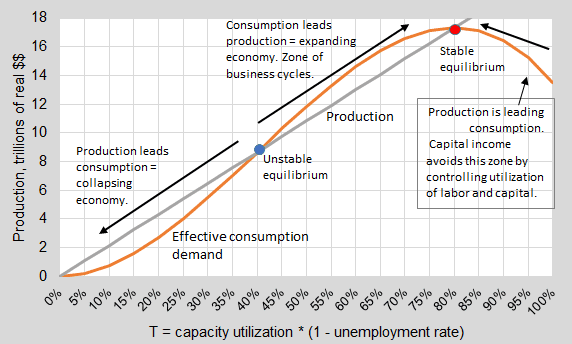The blue dot in this model represents an unstable equilibrium. The economy would seem to want to move away from this blue dot, either toward collapse or balanced growth.

Now the other equation to be integrated is the short-term real interest rate for monetary policy. This equation comes from my effective demand research.

Short-term real rate = z(T2 + L2) – ( 1 – z)*(T + L)

z = (2*L + Natural real rate)/(2L2 + 2L)

Both equations are also based on T and L, but this short-term real rate one incorporates the natural real rate.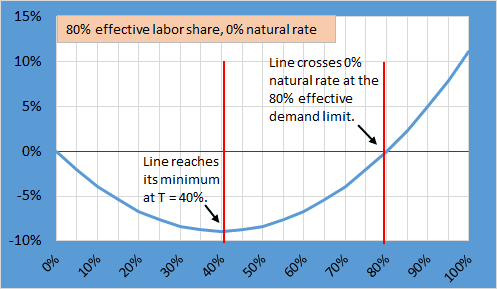Does it make sense that the real rate would start rising below a T of 40%? Shouldn’t the real rate just keep falling to support the economy at lower and lower rates of resource utilization? We need to integrate the above two models to answer that question.

Notice that the minimum of the short-term real rate at 40% matches the unstable equilibrium in the model of Effective Demand.

Let me put them both on the same graph so that you can see. I put production on the left axis and the interest rate on the right axis.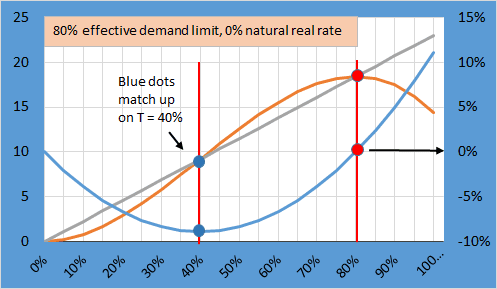Now we can answer the question… Why would the short-term real rate rise going below 40%?… The idea would be to lead the economy toward the unstable equilibrium by lowering rates going from 0% to 40%.  The central bank says, “The more you use labor and capital, we will lower rates to counteract the force pushing the economy away from the unstable equilibrium toward collapse at 0%. ”

Once on the right side of the unstable equilibrium, the short-term real rate can start to rise slowly toward the natural effective demand limit. (80% in the graph above.) At the natural limit, the short-term real rate would be 0% representing a normalized policy rate from a central bank.

The graph above is based on a natural real rate of economic growth of 0%. But what if the economy was growing at 5% or shrinking at -5%? What would happen to the graph? Let’s look at an economy growing at 5%.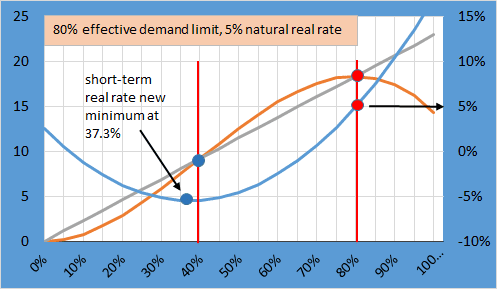The blue dots do not match up now. The short-term real rate has a new minimum at 37.3%. Now what do we do?

We look at the equation for the Effective Demand limit and see the coefficient of e. We can use that coefficient to calibrate the ED limit equation for the natural real rate. Even though the most precise equation would be a very flat quadratic, a sufficient equation is linear…

e = 3.3*natural real rate + 3

So for a 5% natural real rate, the coefficient e would adjust to… 3.3*0.05+3 = 3.165.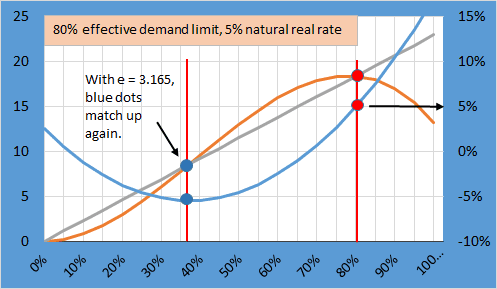So with just a little change in the e coefficient, the effective demand equation adjusts to the short-term real rate equation. Red dots still match up too.

Now the effective demand equation also incorporates the natural real rate. That is an advancement in the theory.

Happy harmony!

Comments Off on Real Rate Integration with Model of Effective Demand | |